Maths PYP Planners

After years and years of experimenting- loads of failures and gradually more and more successes, I am finally feeling like I am creating coherent PYP maths planners that allow a balance between skills needed to be investigated and which allow for student-led enquiries.

Here are some of the more successful planners I've experimented with:

Measuring Time: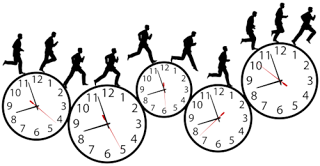Image from: bpritchett.blogspot.com

By understanding astronomy, we have been able to create ways to measure time.

Lines of Inquiry:

• CONNECTION: How the measurement of time is connected to astronomy
• FUNCTION: How our measurement of time system works
• CAUSATION: How our measurement of time system was created
• CONNECTION: Relationships between 12 and 24 hr clocks
• FUNCTION: How to measure elapsed time
• FUNCTION: How we use world time zones

_________________________________________________________________________

Measuring Angles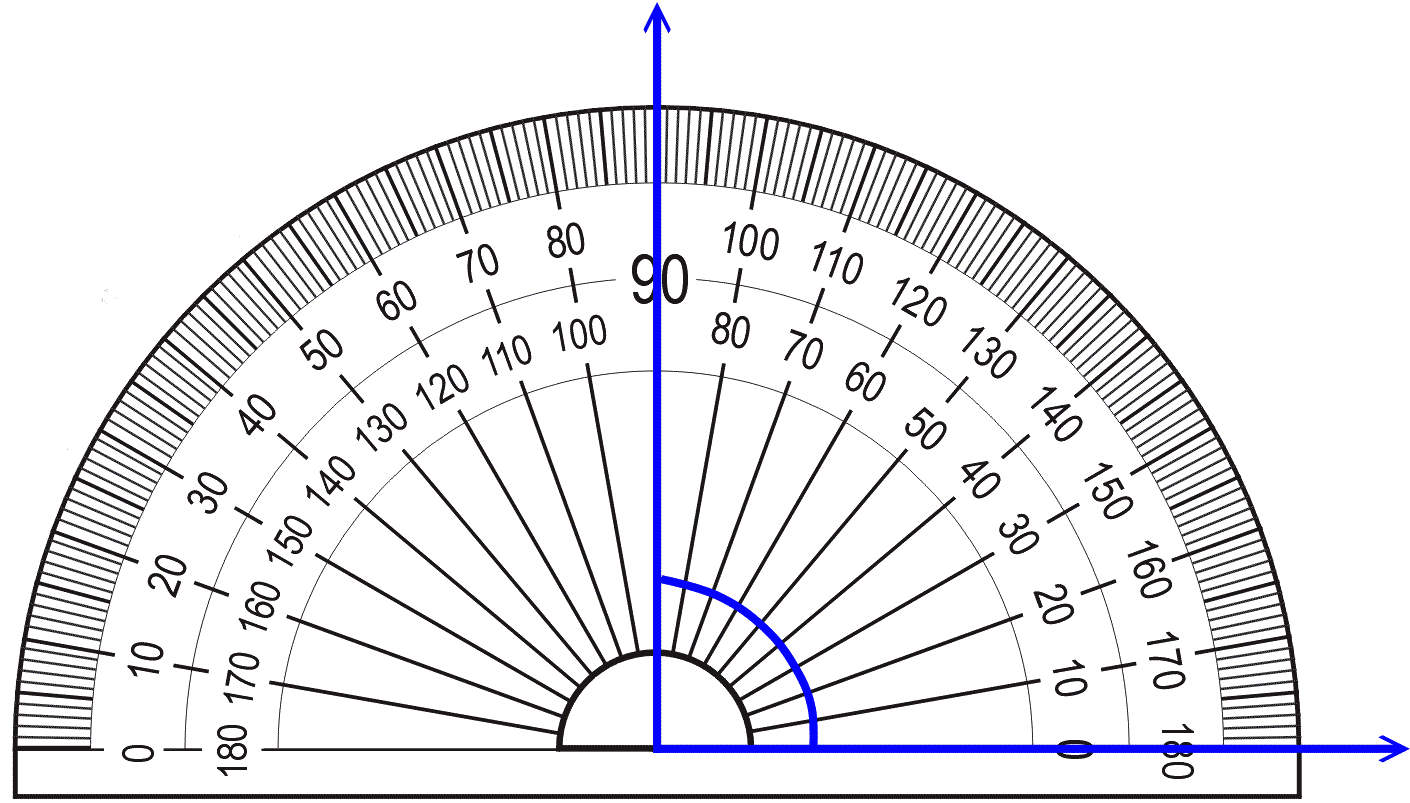Central Idea:

When angles co-exist, connections and relationships form.

Lines of Inquiry:

• FORM: What different types of angles, triangles and quadrilaterals are like
• FUNCTION: How we can estimate & measure the size of angles
• CONNECTION: Patterns and relationships that exist between angles
• FUNCTION: How we use angles in our daily lives

_________________________________________________________________________Lines of Inquiry:

• CONNECTION: The relationship between addition and subtraction
• FUNCTION: How addition/subtraction mental strategies work
• REFLECTION: Determining which mental strategy to use

_________________________________________________________________________

Measuring Area & PerimeterImage from: www.mathgoodies.com

People need to measure the area of space and the length of perimeters for a variety of reasons.

Lines of Inquiry:

• CONNECTION: How area and perimeter are related
• FUNCTION: How to determine the perimeter of complex shapes with unknown lengths
• FUNCTION: How to measure the area of complex shapes
• CAUSATION: Reasons we need to measure perimeter & area in real life

_________________________________________________________________________

Fractions / Decimals / PercentagesImage from: www.mathx.net

We can use fractions, decimals and percentages in a variety of ways in real life situations.

Lines of Inquiry:

• CONNECTION: How fractions, decimals and percentages are related
• CONNECTION: How we use fractions, decimals and percentages in our daily lives
• FUNCTION: Strategies we can use when manipulating fractions, decimals or percentages
• FUNCTION: How we can identify equivalent fractions

_________________________________________________________________________

Data HandlingImage from: www.proprofs.com

Determining the suitability of graphs can help us to investigate and present data more easily.

Lines of Inquiry:

• CAUSATION: Why we use graphs
• FUNCTION: Determining the mean, mode, range, and median of data
• FUNCTION: Interpreting data from a variety of types of graphs
• FUNCTION: Converting data to a percentage to create pie graphs
• REFLECTION: Determining suitability of types of graphs to represent data

_________________________________________________________________________

Measuring Length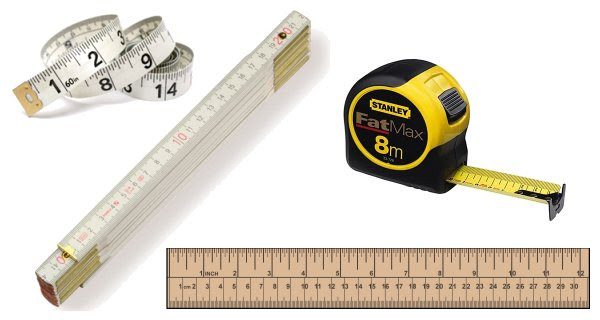Image from:

The metric system for measuring length connects with our base 10 number system.

Lines of Inquiry:

• FORM: The metric v imperial units of measurement for length
• CAUSATION: Why the metric system was created
• FUNCTION: How to measure length using decimals
• FUNCTION: How to convert metric units of length using decimals

_________________________________________________________________________

Chance / Probability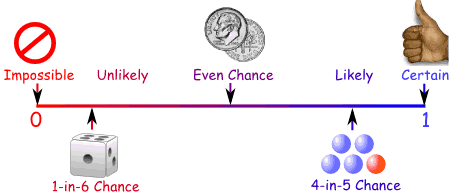Image from: https://www.mathsisfun.com/data/probability.html

Instead of luck, mathematicians think of probability.

Lines of Inquiry:

• CONNECTION: How we use the measurement of probability in our real lives
• FUNCTION: Strategies we can use to measure probability
• CAUSATION: Why we use fractions / decimals / % to measure probability

_________________________________________________________________________

Place Value / Number SystemsImage from:

By understanding the properties of numbers, we can discover patterns and relationships.

Lines of Inquiry:

• FORM:  How positive and negative place values of numbers are infinite
• CAUSATION: Why we can multiply/divide numbers easily by 10, 100, 1000 etc
• CONNECTION: Number properties and their relationships
• CAUSATION:  Reasons we round numbers as a helpful strategy
• CONNECTION: How our Hindi-Arabic number system connects with other number systems

_________________________________________________________________________

Ratios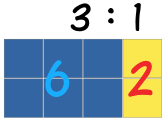Image from: http://www.mathsisfun.com/numbers/ratio.html

We use ratios to help solve real life situations.

Lines of Inquiry:

• FORM: What ratios and proportions are like
• FUNCTION: Strategies we can use to calculate ratio relationships
• CONNECTION: Ways we use ratios in real life situation

______________________________________________________________________

ShapesImage from: www.aspexsoftware.com

Shapes have close or distant relationships with each other.

Lines of Inquiry:

• CONNECTION: Similar properties shapes share
• FUNCTION: Determining the properties of shapes
• CAUSATION: Why shapes have their properties

1.2.